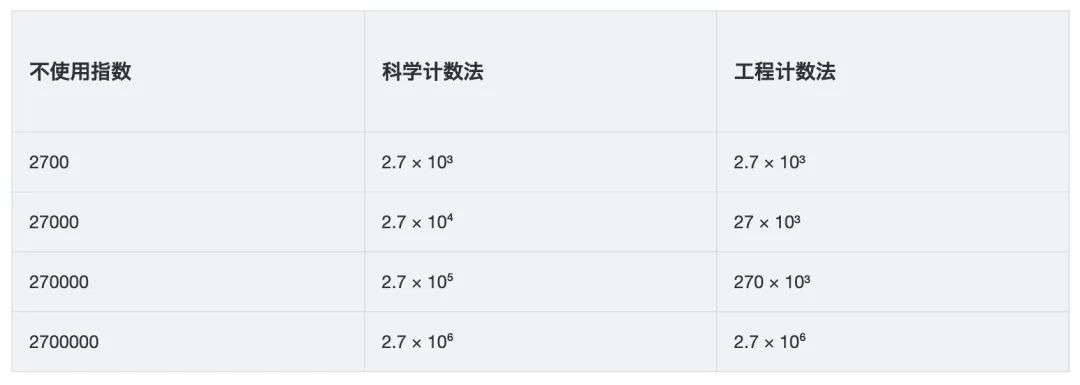# 不掌握这些坑，你敢用BigDecimal吗？

0收藏

BigDecimal概述
Java在java.math包中提供的API类BigDecimal，用来对超过16位有效位的数进行精确的运算。双精度浮点型变量double可以处理16位有效数，但在实际应用中，可能需要对更大或者更小的数进行运算和处理。

BigDecimal对象提供了传统的+、-、*、/等算术运算符对应的方法，通过这些方法进行相应的操作。BigDecimal都是不可变的（immutable）的， 在进行每一次四则运算时，都会产生一个新的对象 ，所以在做加减乘除运算时要记得要保存操作后的值。

BigDecimal的4个坑

`````` @Test
public void test0(){
float a = 1;
float b = 0.9f;
System.out.println(a - b);
}``````

`````` @Test
public void test1(){
BigDecimal a = new BigDecimal(0.01);
BigDecimal b = BigDecimal.valueOf(0.01);
System.out.println("a = " + a);
System.out.println("b = " + b);
}``````

``````a = 0.01000000000000000020816681711721685132943093776702880859375
b = 0.01``````

``````    public static BigDecimal valueOf(double val) {
// Reminder: a zero double returns '0.0', so we cannot fastpath
// to use the constant ZERO.  This might be important enough to
// justify a factory approach, a cache, or a few private
// constants, later.
return new BigDecimal(Double.toString(val));
}``````

``````BigDecimal(int)       创建一个具有参数所指定整数值的对象。
BigDecimal(double)    创建一个具有参数所指定双精度值的对象。
BigDecimal(long)      创建一个具有参数所指定长整数值的对象。
BigDecimal(String)    创建一个具有参数所指定以字符串表示的数值的对象。``````

``````@Test
public void test2(){
BigDecimal a = new BigDecimal("0.01");
BigDecimal b = new BigDecimal("0.010");
System.out.println(a.equals(b));
System.out.println(a.compareTo(b));
}``````

equals方法是基于BigDecimal实现的equals方法来进行比较的，直观印象就是比较两个对象是否相同，那么代码是如何实现的呢？

`````` @Override
public boolean equals(Object x) {
if (!(x instanceof BigDecimal))
return false;
BigDecimal xDec = (BigDecimal) x;
if (x == this)
return true;
if (scale != xDec.scale)
return false;
long s = this.intCompact;
long xs = xDec.intCompact;
if (s != INFLATED) {
if (xs == INFLATED)
xs = compactValFor(xDec.intVal);
return xs == s;
} else if (xs != INFLATED)
return xs == compactValFor(this.intVal);

return this.inflated().equals(xDec.inflated());
}``````

`````` @Test
public void test3(){
BigDecimal a = new BigDecimal("1.0");
BigDecimal b = new BigDecimal("3.0");
a.divide(b);
}``````

``````java.lang.ArithmeticException: Non-terminating decimal expansion; no exact representable decimal result.

at java.math.BigDecimal.divide(BigDecimal.java:1690)
...``````

If the quotient has a nonterminating decimal expansion and the operation is specified to return an exact result, an ArithmeticException is thrown. Otherwise, the exact result of the division is returned, as done for other operations.

`````` @Test
public void test3(){
BigDecimal a = new BigDecimal("1.0");
BigDecimal b = new BigDecimal("3.0");
BigDecimal c = a.divide(b, 2,RoundingMode.HALF_UP);
System.out.println(c);
}``````

● RoundingMode.UP：舍入远离零的舍入模式。在丢弃非零部分之前始终增加数字(始终对非零舍弃部分前面的数字加1)。注意，此舍入模式始终不会减少计算值的大小。
● RoundingMode.DOWN：接近零的舍入模式。在丢弃某部分之前始终不增加数字(从不对舍弃部分前面的数字加1，即截短)。注意，此舍入模式始终不会增加计算值的大小。
● RoundingMode.CEILING：接近正无穷大的舍入模式。如果 BigDecimal 为正，则舍入行为与 ROUNDUP 相同;如果为负，则舍入行为与 ROUNDDOWN 相同。注意，此舍入模式始终不会减少计算值。
● RoundingMode.FLOOR：接近负无穷大的舍入模式。如果 BigDecimal 为正，则舍入行为与 ROUNDDOWN 相同;如果为负，则舍入行为与 ROUNDUP 相同。注意，此舍入模式始终不会增加计算值。
● RoundingMode.HALF_UP：向“最接近的”数字舍入，如果与两个相邻数字的距离相等，则为向上舍入的舍入模式。如果舍弃部分 >= 0.5，则舍入行为与 ROUND_UP 相同;否则舍入行为与 ROUND_DOWN 相同。注意，这是我们在小学时学过的舍入模式(四舍五入)。
● RoundingMode.HALF_DOWN：向“最接近的”数字舍入，如果与两个相邻数字的距离相等，则为上舍入的舍入模式。如果舍弃部分 > 0.5，则舍入行为与 ROUND_UP 相同;否则舍入行为与 ROUND_DOWN 相同(五舍六入)。
● RoundingMode.HALF_EVEN：向“最接近的”数字舍入，如果与两个相邻数字的距离相等，则向相邻的偶数舍入。如果舍弃部分左边的数字为奇数，则舍入行为与  ● ROUNDHALFUP 相同;如果为偶数，则舍入行为与 ROUNDHALF_DOWN 相同。注意，在重复进行一系列计算时，此舍入模式可以将累加错误减到最小。此舍入模式也称为“银行家舍入法”，主要在美国使用。四舍六入，五分两种情况。如果前一位为奇数，则入位，否则舍去。以下例子为保留小数点1位，那么这种舍入方式下的结果。1.15 ==> 1.2 ,1.25 ==> 1.2
● RoundingMode.UNNECESSARY：断言请求的操作具有精确的结果，因此不需要舍入。如果对获得精确结果的操作指定此舍入模式，则抛出ArithmeticException。

``````@Test
public void test4(){
BigDecimal a = BigDecimal.valueOf(35634535255456719.22345634534124578902);
System.out.println(a.toString());
}``````

``````3.563453525545672E+16
``````

● toPlainString()：不使用任何科学计数法；
● toString()：在必要的时候使用科学计数法；
● toEngineeringString() ：在必要的时候使用工程计数法。类似于科学计数法，只不过指数的幂都是3的倍数，这样方便工程上的应用，因为在很多单位转换的时候都是10^3；``````NumberFormat currency = NumberFormat.getCurrencyInstance(); //建立货币格式化引用
NumberFormat percent = NumberFormat.getPercentInstance();  //建立百分比格式化引用
percent.setMaximumFractionDigits(3); //百分比小数点最多3位

BigDecimal loanAmount = new BigDecimal("15000.48"); //金额
BigDecimal interestRate = new BigDecimal("0.008"); //利率
BigDecimal interest = loanAmount.multiply(interestRate); //相乘

System.out.println("金额:\t" + currency.format(loanAmount));
System.out.println("利率:\t" + percent.format(interestRate));
System.out.println("利息:\t" + currency.format(interest));``````

``````金额: ￥15,000.48